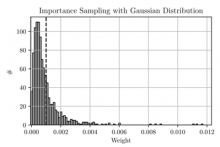Prediction and Evaluation in College Hockey using the Bradley-Terry-Zermelo Model
By John T. Whelan, Adam Wodon
Published in Mathematics for Applications 8, 131 (Sunday, September 1, 2019)

## Abstract

We describe the application of the Bradley-Terry model to NCAA Division I Men's Ice Hockey. A Bayesian construction gives a joint posterior probability distribution for the log-strength parameters, given a set of game results and a choice of prior distribution. For several suitable choices of prior, it is straightforward to find the maximum a posteriori point (MAP) and a Hessian matrix, allowing a Gaussian approximation to be constructed. Posterior predictive probabilities can be estimated by 1) setting the log-strengths to their MAP values, 2) using the Gaussian approximation for analytical or Monte Carlo integration, or 3) applying importance sampling to re-weight the results of a Monte Carlo simulation. We define a method to evaluate any models which generate predicted probabilities for future outcomes, using the Bayes factor given the actual outcomes, and apply it to NCAA tournament results. Finally, we describe an on-line tool which currently estimates probabilities of future results using MAP evaluation and describe how it can be refined using the Gaussian approximation or importance sampling.

Whelan, John T.